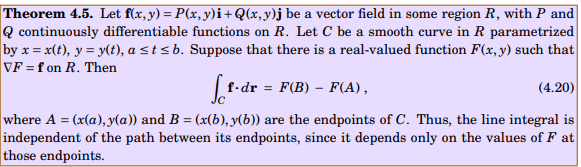# Proving $\displaystyle\int_C \vec{F}\cdot d\vec{r} = 0$ with Closed Curve

• MHB
• WMDhamnekar
In summary, the conversation discusses the proposition that if C is a simple closed curve in the xy plane that does not enclose the origin, then the line integral of a vector field F, given by $\vec{F} = \frac{yi + xj}{x^2 + y^2}$, along C is equal to 0. However, it is proven that this proposition is incorrect as the curl of $\vec{F}$ is nonzero. A potential function is defined for a conservative vector field, and it is shown that $\vec{F}$ does have a potential function. It is also shown through a concrete counterexample that the line integral along any curve C in the given example is equal to 0. The conversationf

#### WMDhamnekar

MHB
If C is the simple closed curve in the xy plane not enclosing the origin, how to prove that $\displaystyle\int_C \vec{F}\cdot d\vec{r} =0$ where $$F= \frac{yi +xj}{x^2+y^2}$$

How to answer this question? Any math help will be accepted. I am working on this question. If any member of Math help board know the correct answer, may reply.

This proposition is incorrect, since the curl of $\vec{F}$ is nonzero. For a concrete counterexample, let C be the positively oriented square in the plane with vertices $(1,0), (2,0), (2,1)$, and $(1,1)$. A direct computation shows $\int_C \vec{F}\cdot d\vec{r} ≠ 0$.

This proposition is incorrect, since the curl of $\vec{F}$ is nonzero. For a concrete counterexample, let C be the positively oriented square in the plane with vertices $(1,0), (2,0), (2,1)$, and $(1,1)$. A direct computation shows $\int_C \vec{F}\cdot d\vec{r} ≠ 0$.A real valued function F(x,y) such that $\nabla {F(x,y)}=f(x,y)$ is called potential for f. A conservative vector field is one which has a potential.

Now, in our problem, we have to show that $\vec{F} =\frac{yi +xj}{x^2+y^2}$ is a conservative vector field (It has a potential).
Thus, a potential $\mathcal{F(x,y)}$ for $F(x,y) = \frac{yi}{x^2+y^2} + \frac{xj}{x^2+y^2}$ exists, namely,
$$\arctan{(\frac{x}{y})} + \arctan{(\frac{y}{x})}.$$

You can verify in your example that the value of line integral F along any curve C going from (1,0) to (2,0) or from (2,0) to (2,1) or from (2,1) to (1,1) and finally from (1,1) to (1,0) will always be zero. Since by above given theorem
$$\displaystyle\int_C\vec{F}\cdot d\vec{r} = \mathcal{F} (2,0) - \mathcal{F} (1,0) = \arctan{(\frac20)} + \arctan{(\frac02)} - \arctan{(\frac10)}-\arctan{(\frac01)} = \frac{\pi}{2} -\frac{\pi}{2} =0$$

View attachment 11853
A real valued function F(x,y) such that $\nabla {F(x,y)}=f(x,y)$ is called potential for f. A conservative vector field is one which has a potential.

Now, in our problem, we have to show that $\vec{F} =\frac{yi +xj}{x^2+y^2}$ is a conservative vector field (It has a potential).
Thus, a potential $\mathcal{F(x,y)}$ for $F(x,y) = \frac{yi}{x^2+y^2} + \frac{xj}{x^2+y^2}$ exists, namely,
$$\arctan{(\frac{x}{y})} + \arctan{(\frac{y}{x})}.$$

You can verify in your example that the value of line integral F along any curve C going from (1,0) to (2,0) or from (2,0) to (2,1) or from (2,1) to (1,1) and finally from (1,1) to (1,0) will always be zero. Since by above given theorem
$$\displaystyle\int_C\vec{F}\cdot d\vec{r} = \mathcal{F} (2,0) - \mathcal{F} (1,0) = \arctan{(\frac20)} + \arctan{(\frac02)} - \arctan{(\frac10)}-\arctan{(\frac01)} = \frac{\pi}{2} -\frac{\pi}{2} =0$$
A potential function exists if and only if the curl of the vector field is zero. Here we have $$\displaystyle \nabla \times \left ( \dfrac{y}{x^2 + y^2} \hat{i} + \dfrac{x}{x^2 + y^2} \hat{j} \right ) = - \dfrac{2 (x^2 - y^2)}{(x^2 + y^2)^2} \hat{k}$$.

-Dan

A potential function exists if and only if the curl of the vector field is zero. Here we have $$\displaystyle \nabla \times \left ( \dfrac{y}{x^2 + y^2} \hat{i} + \dfrac{x}{x^2 + y^2} \hat{j} \right ) = - \dfrac{2 (x^2 - y^2)}{(x^2 + y^2)^2} \hat{k}$$.

-Dan
$\nabla \times \bigg(\frac{y}{x^2+y^2}, \frac{x}{x^2+y^2},0\bigg)= \begin{vmatrix} i & j & k \\ \frac{\partial}{\partial{x}} & \frac{\partial}{\partial{y}} & \frac{\partial}{\partial{z}} \\ \frac{y}{x^2+y^2} & \frac{x}{x^2+y^2} & 0 \end{vmatrix} = \hat{i} (0 - 0) +\hat{j} (0 - 0) + \hat{k} \bigg(\frac{-2xy}{(x^2+y^2)^2} -\frac{-2xy}{(x^2+y^2)^2}\bigg)= 0$

My computation of curl of conservative vector field is zero. How is that?(Bigsmile)(Clapping)

Last edited:
$\nabla \times \bigg(\frac{y}{x^2+y^2}, \frac{x}{x^2+y^2},0\bigg)= \begin{vmatrix} i & j & k \\ \frac{\partial}{\partial{x}} & \frac{\partial}{\partial{y}} & \frac{\partial}{\partial{z}} \\ \frac{y}{x^2+y^2} & \frac{y}{x^2+y^2} & 0 \end{vmatrix} = \hat{i} (0 - 0) +\hat{j} (0 - 0) + \hat{k} \bigg(\frac{-2xy}{(x^2+y^2)^2} -\frac{-2xy}{(x^2+y^2)^2}\bigg)= 0$

My computation of curl of conservative vector field is zero. How is that?(Bigsmile)(Clapping)
A typo? Check the (3,2) component.
\begin{vmatrix} i & j & k \\ \frac{\partial}{\partial{x}} & \frac{\partial}{\partial{y}} & \frac{\partial}{\partial{z}} \\ \frac{y}{x^2+y^2} & \frac{x}{x^2+y^2} & 0 \end{vmatrix}

$$\displaystyle \dfrac{ \partial }{ \partial x} \dfrac{x}{x^2 + y^2} - \dfrac{ \partial }{ \partial y} \dfrac{y}{x^2 + y^2} = \dfrac{((x^2 + y^2) - x(2x) ) - ((x^2 + y^2) - y(2y) }{ (x^2 + y^2)^2} = -2 \dfrac{x^2 - y^2}{(x^2 + y^2)^2}$$

-Dan

Last edited by a moderator:
A typo? Check the (3,2) component.
\begin{vmatrix} i & j & k \\ \frac{\partial}{\partial{x}} & \frac{\partial}{\partial{y}} & \frac{\partial}{\partial{z}} \\ \frac{y}{x^2+y^2} & \frac{x}{x^2+y^2} & 0 \end{vmatrix}

-Dan
Thanks. I corrected that now. You are correct. Curl of $\vec{f}$ is nonzero.

Last edited: